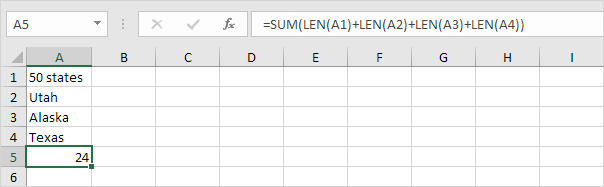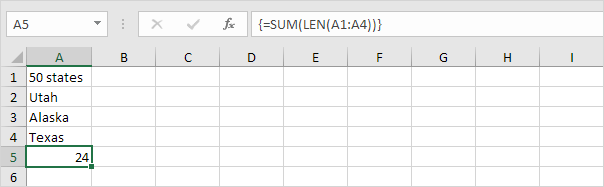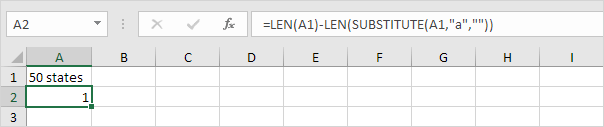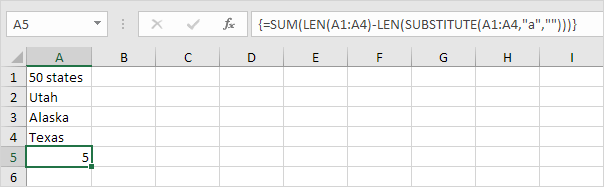# Count Characters

Learn how to count the number of characters in a cell or range of cells and how to count how many times a specific character occurs in a cell or range of cells.

1. The LEN function in Excel counts the number of characters in a cell.Explanation: the LEN function counts 2 numbers, 1 space and 6 letters.

2. Use SUM and LEN to count the number of characters in a range of cells.Note: as you can imagine, this formula can get quite long.

3. The array formula below counts the number of characters in a range of cells.Note: finish an array formula by pressing CTRL + SHIFT + ENTER. Excel adds the curly braces {}. The array constant {9;4;6;5} is used as an argument for the SUM function, giving a result of 24. Maybe this is one step too far for you at this stage, but it shows you one of the many other powerful features Excel has to offer.

4. Use LEN and SUBSTITUTE to count how many times a specific character (in this example, the character a) occurs in a cell.Explanation: the SUBSTITUTE function replaces the character a (second argument) with an empty string (third argument). LEN(SUBSTITUTE(A1,”a”,””)) equals 8 (the length of the string without the character a). If we subtract this number from 9 (total number of characters in cell A1), we get the number of occurrences of the character a in cell A1.

5. The array formula below counts how many times a specific character (in this example, the character a) occurs in a range of cells.Note: finish an array formula by pressing CTRL + SHIFT + ENTER. Excel adds the curly braces {}. The array constant {1;1;2;1} is used as an argument for the SUM function, giving a result of 5. The SUBSTITUTE function is case-sensitive. The A in Alaska is not counted.

6. The array formula below counts both lower and upper case occurrences of a specific character (in this example, the character a).Explanation: The LOWER function converts all letters to lowercase first.

Previous articleCount Blank/Nonblank Cells in Excel
Next articleDynamic Named Range in Excel